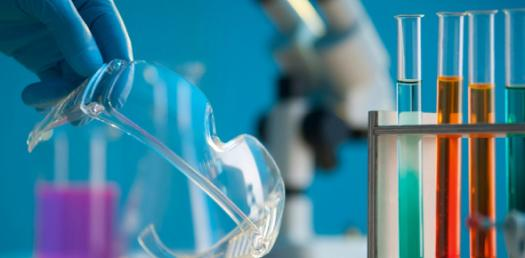# Can You Answer These Chemistry Questions? Hardest Trivia Quiz

35 Questions | Total Attempts: 305Settings.

• 1.
What is the atomic number of fluorine?
• A.

8

• B.

9

• C.

10

• D.

18

• E.

19

• 2.
Carbon-14 (carbon with an atomic mass of 14) is a radioactive isotope used to determine the age of objects composed of organic matter. Carbon-12 and Carbon-13 are other isotopes of carbon. The number of which of the following is different among these 3 carbon isotopes?
• A.

Electron

• B.

Neutron

• C.

Proton

• D.

Photon

• 3.
Lead (Pb-208), lead with an atomic mass of 208, is the heaviest stable isotope known. It has an atomic number of 82.  How many neutrons does Pb-208 have?
• A.

82

• B.

126

• C.

164

• D.

208

• 4.
Chlorine with a charge of -1 has how many electrons?
• A.

16

• B.

17

• C.

18

• D.

35

• E.

36

• 5.
How many protons does Chlorine have
• A.

17

• B.

18

• C.

35

• D.

36

• 6.
What is the atomic mass for Chlorine?
• A.

17

• B.

18

• C.

35

• D.

36

• 7.
In glycerol (C3H8O3), there is a hydroxyl group (OH) bound to each of the 3 carbon atoms. The bond between the oxygen atom of a hydroxyl group and a carbon atom (C-O bond)  in glycerol would be which type of bond?
• A.

Covalent

• B.

Ionic

• C.

Hydrogen

• D.

James

• 8.
Li (Lithium) and F (Fluorine) would most likely form which kind of bond?
• A.

Covalent

• B.

Ionic

• C.

Hydrogen

• D.

James

• 9.
What are the three particles that make up atoms?
• A.

Protons, neutrons, and isotopes

• B.

Neutrons, isotopes, and electrons

• C.

Positives, negatives, and neutrals

• D.

Neutrons, electrons, and protons

• 10.
What are found in the space surrounding the nucleus of an atom?
• A.

Protons

• B.

Electrons

• C.

Neutrons

• D.

Ions

• 11.
Breaking which type of bond would require the most energy?
• A.

Covalent

• B.

Hydrogen

• C.

Ionic

• D.

James

• 12.
Isotopes are atoms of the same element with the same number of protons and a different number of ___.
• A.

Electrons

• B.

Molecules

• C.

Neutrons

• D.

Ions

• 13.
Which type of ion forms when an atom gains an electron?
• A.

Neutral

• B.

Positive

• C.

Negative

• D.

• 14.
What is an ion?
• A.

An atom with a different number of protons and neutrons.

• B.

An atom with a different number of protons and electrons.

• C.

An atom with a different number of electrons and neutrons.

• D.

An atom with the same number of protons and electrons.

• 15.
A map of eastern North America, showing the pH of rainfall in the various states, indicates that the pH of rain in New York state varies from 4.22 to 4.40.  According to these figures, the most acidic rainfall in New York state has a pH of ___.
• A.

4.22

• B.

4.30

• C.

4.35

• D.

4.40

• 16.
Which of the following has the greatest concentration of H+ ions?
• A.

Baking soda, pH of 9.0

• B.

Milk of Magnesia, pH of 10.5

• C.

Ammonia, pH of 11.5

• D.

Bleach, pH of 12.8

• 17.
Which of the following has the greatest concentration of OH - ions?
• A.

Urine, pH 6.0

• B.

Rainwater, pH 5.5

• C.

Tomato juice, pH 4.0

• D.

Gastric juice, pH 2.0

• 18.
In a water molecule, shared electrons spend more time around the oxygen atom than the hydrogen atoms. As a result, the oxygen atom is?
• A.

Very negative

• B.

Slightly positive

• C.

Slightly negative

• D.

All of the above

• 19.
What bonds are formed by water molecules attracting to other water molecules through a process called cohesion?
• A.

James

• B.

Covalent

• C.

Ionic

• D.

Hydrogen

• 20.
Which of the following chemical formulas represents an organic molecule?
• A.

Silver Nitrate, AgNO3

• B.

Water, H2O

• C.

Disaccharide, C12H22O11

• D.

Ammonia, NH3

• 21.
What is the monomer of nucleic acids?
• A.

Monosaccharides

• B.

Nucleotides

• C.

Fatty acids

• D.

Amino acids

• 22.
How do enzymes speed up chemical reactions?
• A.

Enzymes decrease the activation energy.

• B.

Enzymes decrease the energy produced by the reaction.

• C.

Enzymes increase the activation energy.

• D.

Enzymes increase the energy produced by the reaction.

• 23.
An amino acid is the monomer of which of the following macromolecules?
• A.

Carbohydrate

• B.

Lipid

• C.

Nucleic Acid

• D.

Protein

• 24.
The image depicts the monomer for which of the following molecules?
• A.

Carbohydrate

• B.

Lipid

• C.

Nucleic Acid

• D.

Protein

• 25.
This image depicts a single glucose molecule. What would it be classified as?
• A.

Monosaccharide

• B.

Disaccharide

• C.

Polysaccharide

• D.

Polymer

Related TopicsBack to top